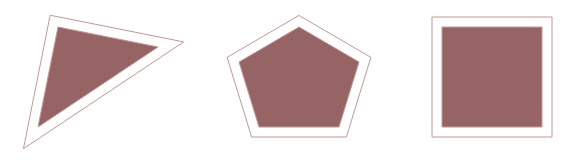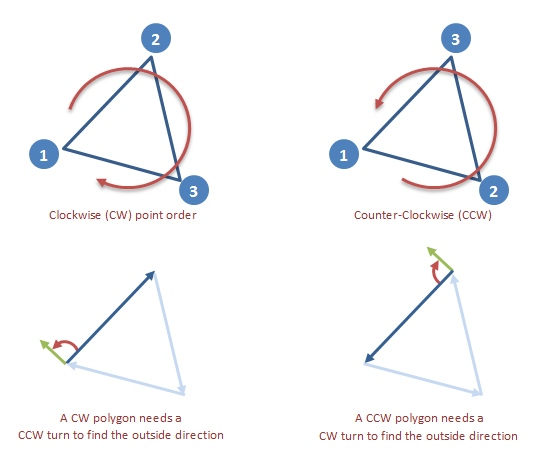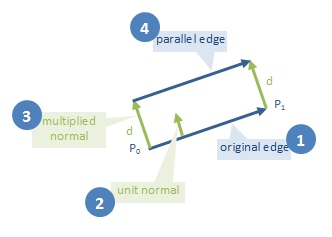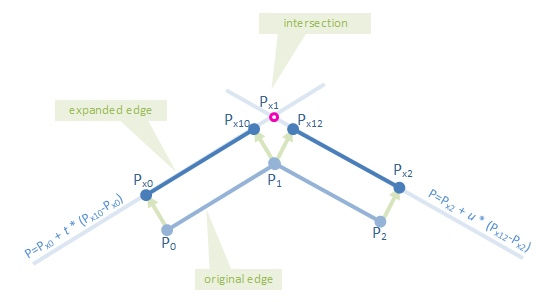# 多边形扩展算法实现

2021/12/01    其他

``````// 由四个顶点构成的多边形
const pointList = [[0, 0], [0, 10], [10, 10], [10, 0]]
// 以上多边形，边缘扩大1后，计算新的顶点坐标集合
const expandWidth = 1
``````

## 算法原理``````type IPoint = [number, number]
type IPolyonPoints = IPoint[]
``````

## 寻找定点``````function vecDot(v1: IPoint, v2: IPoint) {
return v1 * v2 + v1 * v2;
}

function vecRot90CW(v: IPoint): IPoint {
return [v, -v]
}

function vecRot90CCW(v: IPoint): IPoint {
return [-v, v]
}

function polyIsCw(p: IPoint[]) {
return vecDot( vecRot90CW( [ p - p, p - p ]), [ p - p, p - p ]) >= 0;
}

var rot = polyIsCw(p) ? vecRot90CCW : vecRot90CW;
``````

## 找到平行于多边形边的线``````function vecUnit(v: IPoint): IPoint {
var len = Math.sqrt(v * v + v * v);
return [v / len, v / len]
}

function vecMul(v: IPoint, s: number): IPoint {
return [ v * s, v * s ]
}

var v01 = [ pt1 - pt0, pt1 - pt0 ];  // edge vector
var d01 = vecMul(vecUnit(rot(v01)), distance);     // multiplied unit normal
var ptx0 = [ pt0 + d01, pt0 + d01 ]; // two points on the
var ptx1 = [ pt1 + d01, pt1 + d01 ];
``````

## 计算平行线的交点

–这些将是扩展多边形的顶点。``````P = P1 + t * (P2 - P1)
``````

``````P = P1 + t * (P2 - P1)
P = P3 + u * (P4 - P3)
``````

``````P = P1 + t * (P2 - P1) = P3 + u * (P4 - P3)
``````

``````(P2 - P1) * t + (P3 - P4) * u = P3 - P1
``````

``````(P2.x - P1.x) * t + (P3.x - P4.x) * u = P3.x - P1.x
(P2.y - P1.y) * t + (P3.y - P4.y) * u = P3.y - P1.y
``````

``````a1 = P2.x - P1.x    a2 = P2.y - P1.y
b1 = P3.x - P4.x    b2 = P3.y - P4.y
c1 = P3.x - P1.x    c2 = P3.y - P1.y
``````

``````a1*t + b1*u = c1
a2*t + b2*u = c2
``````

``````t = (b1*c2 - b2*c1)/(a2*b1 - a1*b2)
``````

``````function intersect(line1: IPoint[], line2: IPoint[]) {
var a1 = line1 - line1;
var b1 = line2 - line2;
var c1 = line2 - line1;

var a2 = line1 - line1;
var b2 = line2 - line2;
var c2 = line2 - line1;

var t = (b1 * c2 - b2 * c1) / (a2 * b1 - a1 * b2);

return {
x: line1 + t * (line1 - line1),
y: line1 + t * (line1 - line1)
};
}
``````